Sample Lab Previewer

## Lab 4: The Cosmic Distance Ladder I: Parallax

Instructions

Read the lab before watching the summary video. You might find it easier to navigate if you expand only one or two sections at a time.

Watch the summary video before doing the lab:

Cosmic Distance Ladder I: Parallax (29:27)

You may save your work as many times as you like, but you are permitted only one submission per answer box.

Enter all calculated values to at least two significant digits.

1. -
Question part
Points
 1 2 3 4 5 6 7 8 9 10 11 12 13 14 15 16 17 18 19 20 21 22 23 24 25 26 27 28 29 30 31 32 33 34 35 36 37 38 39 40 41 -- -- -- -- -- -- -- -- -- -- -- -- -- -- -- -- -- -- -- -- -- -- -- -- -- -- -- -- -- -- -- -- -- -- -- -- -- -- -- -- --
Total
0/54
LAB 4: COSMIC DISTANCE LADDER I: PARALLAX

• ## GOALS

In this lab, you will:
1. Use parallax to measure distances to objects on Earth.
2. Use parallax and Earth's diameter to measure distances to objects within our solar system.
3. Use parallax measurements of objects within our solar system to measure the astronomical unit (AU).
4. Use parallax and the AU to measure distances to nearby stars.
• ## EQUIPMENT

Protractor with attached string
Tape measure
Computer with internet connection
• ## BACKGROUND: A. THE COSMIC DISTANCE LADDER

Distance is one of the most difficult things to measure in astronomy. You cannot see distance. You never know if you are looking at a low-luminosity star nearby (A) or a high-luminosity star far away (B).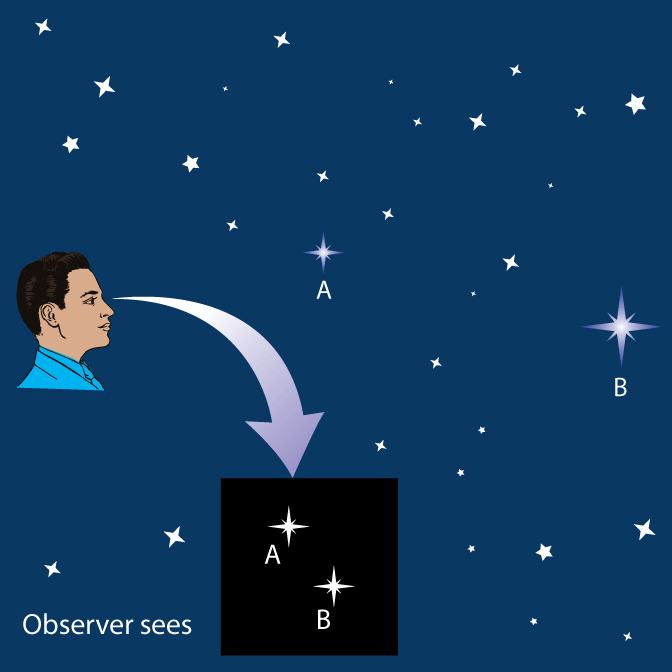In both cases, the star can appear to be equally bright.

To deal with this, astronomers have developed a hierarchy of techniques for measuring greater and greater distances.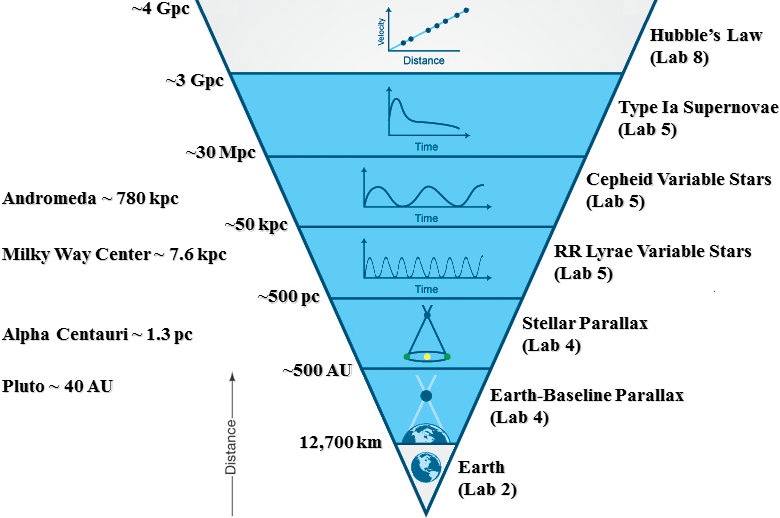In Lab 2, you measured Earth's diameter in kilometers. This is the base of the cosmic distance ladder.

In this lab, you will use Earth's diameter in kilometers and a technique called parallax to measure distances to two solar-system objects: an asteroid and the planet Venus.

You will then use your measurement of the distance to Venus to determine how many kilometers are in one astronomical unit (AU). One AU is the average distance between Earth and the sun.

Finally, you will use your measurement of the AU in kilometers and parallax to measure the distance to the closest star system to the sun: Alpha Centauri.

With current technology, parallax can only be used to measure distances out to about 500 parsecs, which is about 1.5 x 1016 kilometers. This might seem like a great distance, but it is only about 7% of the way to the center of our Milky Way galaxy. In Labs 5 and 8, you will learn techniques that can be used to measure distances across the entire Milky Way galaxy and to other galaxies, both nearby and far away.
• ## BACKGROUND: B. PARALLAX

Hold a pencil vertically in front of and close to your nose. Focus on distant objects or a background wall. Close one eye and then open it while closing the other. Notice how much your pencil appears to shift with respect to the distant objects. This is called parallax: the apparent shift of a foreground object with respect to background objects due only to a change in the observer's position.

Now, hold your pencil at arms length and repeat this exercise. Is the angular shift smaller or larger?

The closer the foreground object, the larger the angular shift. The farther away the foreground object, the smaller the angular shift.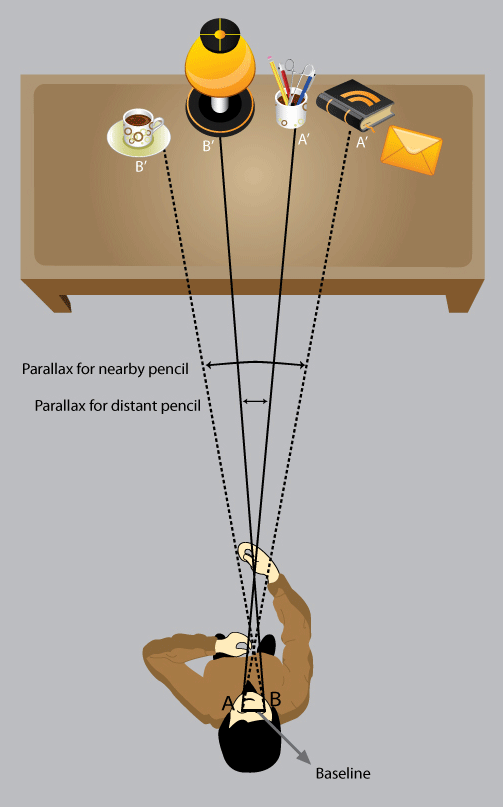The distance between the two observing positions is called the baseline. In the above exercise, the baseline is the distance between your eyes.

Astronomically, two baselines are commonly used.

1. Earth-Baseline Parallax: The two observing positions are different positions on Earth, in which case the baseline is a fraction of Earth's diameter (which you measured in Lab 2).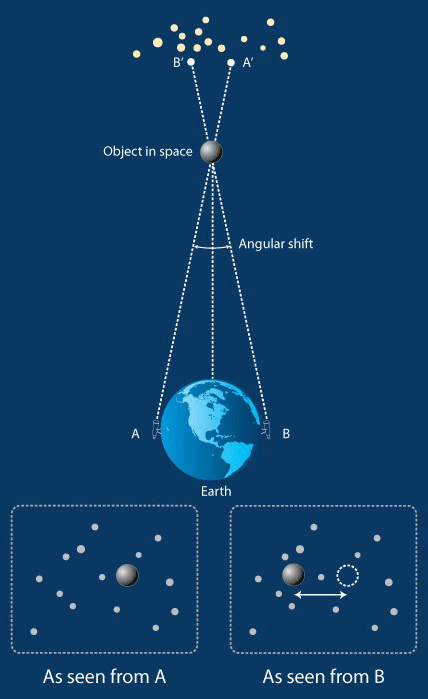2. Stellar Parallax: The two observing positions are on opposite sides of Earth's orbit around the sun, in which case the baseline is two AU (which you will measure in Section C of the procedure of this lab).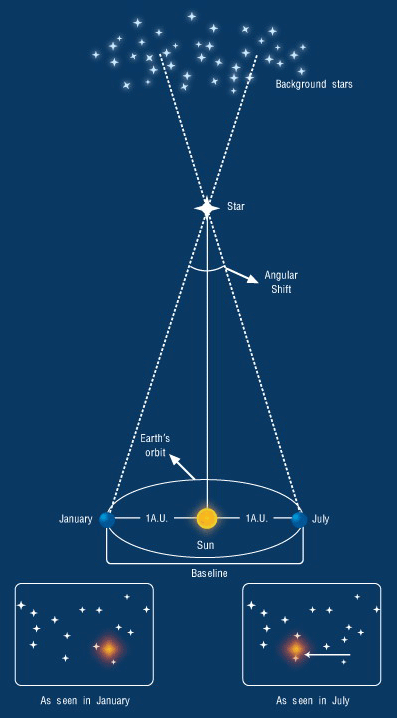In either case, the relationship between the baseline between the two observing positions, the angular shift of the foreground object due to parallax, and the distance to the foreground object is the same.

1. Earth-Baseline Parallax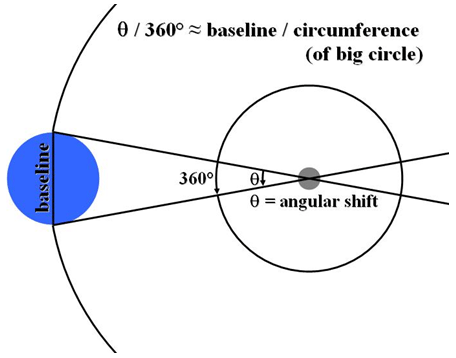2. Stellar Parallax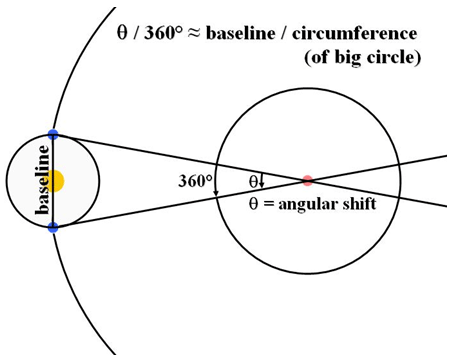The baseline as a fraction of the circumference of the big circle is the same as the angular shift as a fraction of 360 degrees.
 baseline circumference
=  θ 360 deg

Since circumference = 2π times radius and the radius of the big circle is the distance to the foreground object:
 baseline 2π x distance
=  θ 360 deg
.

Solving for the distance to the foreground object yields the following.
distance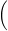baseline 2πx360 deg θNote: Remember to convert θ to degrees before using this equation!

Hence, by (1) knowing the baseline between two observing points, and (2) measuring the angular shift of a foreground object due to parallax, you can (3) measure the distance to the foreground object.

Under ideal circumstances, Earth-baseline parallax can be used to measure distances to objects out to about 500 AU, beyond which the angular shift is too small to be measured accurately given current technology.

However, larger baselines result in larger, and consequently easier to measure, angular shifts. Stellar parallax can be used to measure distances to objects out to about 500 pc, which is about 108 AU.

To measure distances to objects beyond 500 pc, astronomers employ standard-candle techniques, which is the subject of Lab 5.
• ## PROCEDURE: A. DISTANCES TO OBJECTS ON EARTH

We will begin by using parallax to measure distances to two objects here on Earth.

On-campus students at UNC-Chapel Hill will stand behind the south wall of the Chapman Observing Deck and measure distances to two objects in front of them: (1) a smokestack on the roof of Morehead Laboratory (not Morehead Planetarium or Morehead Observatory), and (2) the campus water tower. On-campus students at other institutions will be taken to an analogous locale by their instructor.Note: Online students will need to find a wall, or fence, or similar structure behind which they can stand and over which they can identify two objects to measure distances to. Measure the closer object first and use similar baselines for both measurements.

First, stand on the far left side of the wall. Place your protractor flush against the back of the wall and squat down such that your eyes are right behind where the string attaches to the protractor. Move the string such that it points to the smokestack (the closer of the two objects).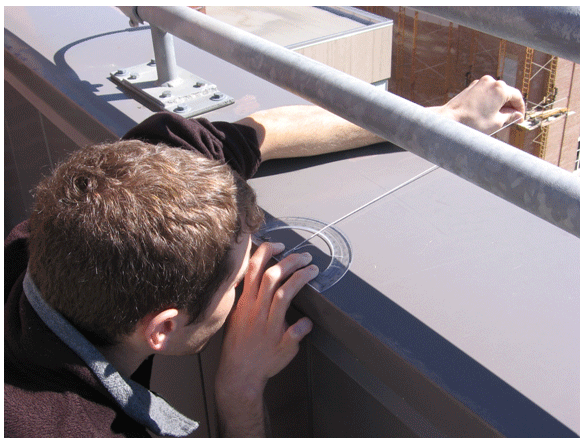Question: Without moving the string, stand up and read the angle off of the protractor to the nearest 0.1 degrees. (1 point)  first angle deg

Question: Mark the position of your protractor on the wall (e.g., with a pebble) and move to the far right side of the wall. Repeat your measurement. (1 point)  second angle deg

The positive value of the difference between these two angles is the angular shift due to parallax.
angular shift = |first angle − second angle|

Question: Record your angular shift. (1 point)
deg

Question: Next, use the tape measure to measure the baseline between your two observing positions in feet. (1 point)
ft

Note: The tape measure will probably not be long enough, in which case measure one part at a time and add the measurements together.

Question: Calculate the distance to the smokestack using parallax. (1 point)
distancebaseline 2πx360 deg θft

The true distance to the smokestack is 360 feet.

Note: On-campus students at other institutions: The true distance to each of your objects will be provided by your instructor.

Note: Online students will need to measure the true distance to each of their objects with the tape measure. Consequently, select objects that are not unreasonably far away (but certainly farther away than the length of your baseline).

Question: Record the true distance to your object in feet. (If your object is the smokestack, enter 360. 1 point)
ft

Question: Calculate your percent error. (1 point)
%

Question: Repeat this exercise for the water tower (the farther of the two objects). The true distance to the water tower is 2800 ft.  Record your results in the following table.

Question: Discuss significant sources of error. (3 points)

Question: Which of your measurements was less accurate? Which should have been less accurate? Why? (2 points)

Question: For a fixed baseline, at what point does parallax cease to be an accurate technique for measuring distance? (Do not say 500 pc.) (2 points)

Question: What are two ways that you could more accurately measure the distance to an object using parallax? (2 points)
• ## PROCEDURE: B. DISTANCE TO AN ASTEROID - 1. Observe an Asteroid with Skynet

First, we are going to try to observe an asteroid with Skynet telescopes in different hemispheres, to measure its distance with parallax.

Since asteroids (and planets) move across the sky quickly, the two telescopes will need to image the asteroid simultaneously. That way, any difference in the asteroid's position between the images will be due to parallax, instead of due to the asteroid moving between when the two images were taken.

This is a very challenging observation to make. First of all, different telescopes will take different amounts of time to slew into position. Consequently, we will shoot a rapid-fire sequence of images from both telescopes. Both telescopes might not start their sequences at the same time, but as long as one image in the first telescope's sequence overlaps in time with one image in the second telescope's sequence, we will be good to go.

This observation is also challenging to make because although the PROMPT telescopes belong to UNC-Chapel Hill, most of Skynet's northern hemisphere telescopes do not. Your priority level is not high enough to override them, but for this week only we will give your instructor a priority level that is high enough to do this. Consequently, we will request only one set of observations per section.

Your instructor will select an asteroid that is observable to both PROMPT and the Skynet telescopes in eastern North America for at least ten minutes each night, this time of year. S/he will then request (1) 15 40-second, open-filter exposures on all available PROMPT telescopes, and (2) 15 40-second, open-filter exposures on all available Skynet telescopes in eastern North America. Finally, for both observations, s/he will set "Don't start before (UTC):" to a time that the asteroid is observable to all of these telescopes.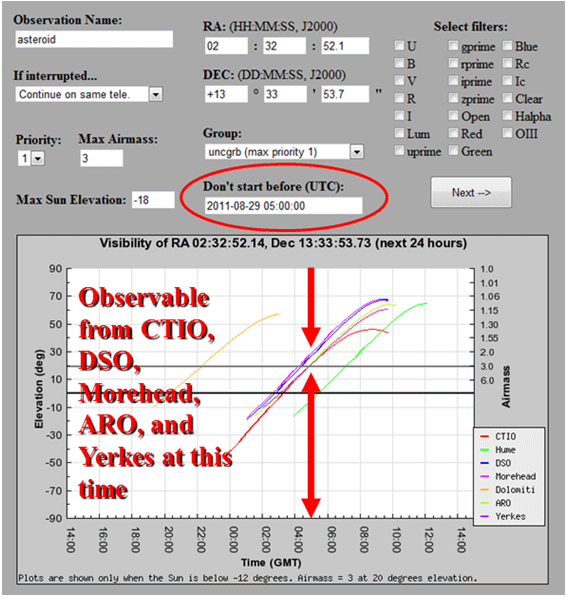If both observations do not go through on the first night (e.g., if a gamma-ray burst goes off, or if all of the telescopes in one hemisphere or the other are clouded out or offline) you instructor will re-request the observations each night until both go through.

You will be able to access these images once they are completed from your instructor's folder under "Sample Images", "Astro 101 Lab", "Lab 4 - Parallax".
• ## PROCEDURE: B. DISTANCE TO AN ASTEROID - 2. Identify the Asteroid

First, use Afterglow to identify which object is the asteroid.
1. Identify which of the two telescopes produced better images.
2. Select and align all of the images from this telescope. For "Alignment Method", select "One Star Manual". For "Cropping Method", select "Intersection". Select "Centroid Sources" and "Stellar Method (PSF)". In your first image, identify a bright, but not saturated, star that is not on the edge of the image. Align to this star in each of the images.
3. Turn your selected and aligned images into a movie. The asteroid will be the moving object, unless you accidentally aligned to the asteroid, in which case everything else in the image will be moving. Download your movie and check it by playing it with other software on your computer.

• ## PROCEDURE: B. DISTANCE TO AN ASTEROID - 3. Measure the Distance to the Asteroid

In this tutorial, you will learn how to select and align images that were taken by different telescopes.

In this tutorial, you will learn (1) how to overlay two images that have already been aligned and (2) how to measure the angular shift of a relatively nearby object in the images due to parallax.

Next, use Afterglow (1) to select, align, and overlay two images of the asteroid that were taken nearly simultaneously by Skynet telescopes in different hemispheres and (2) to measure the angular shift of the asteroid due to parallax.
2. Select and align these images. For "Alignment Method", select "Two Star Manual". For "Cropping Method", select "Intersection". Select "Centroid Sources" and "Stellar Method (PSF)". For alignment, use two stars (1) that are bright, but not saturated, (2) that are not close to one another in the images, and (3) that are in both images. Do not use the asteroid!
3. Overlay your two aligned images and measure the angular shift of the asteroid due to parallax, to the nearest 0.1 arcseconds.

Question: Record the angular shift. (1 point)
arcsec

The baseline between your two observing positions as a fraction of Earth's diameter is given below.

Baseline Between CTIO And Fraction of Earth's Diameter
Astronomical Research Observatory, IL 0.58
Dark Sky Observatory, NC 0.55
Yerkes Observatory, WI 0.60

Question: Record the fraction of Earth's diameter between your two observing positions. (1 point)

Question: You measured Earth's diameter in Lab 2. Enter the value that you measured. (1 point)
km

Question: Record the true value of Earth's diameter. (1 point)
km

Question: Calculate the baseline between the two observing positions using Earth's true diameter. (1 point)
baseline = fraction of diameter x true diameter
km

Question: Calculate the distance to the asteroid using parallax. (2 points)
distancebaseline 2πx360 deg θNote: Remember to convert θ to degrees before using this equation!
km

Question: Main-belt asteroids are typically between 1.6 x 108 km and 6.4 x 108 km from Earth. Given this, is your measurement reasonable? Discuss significant sources of error. (2 points)
• ## PROCEDURE: C. DISTANCE TO VENUS AND THE SIZE OF THE AU

To measure distances to nearby stars with stellar parallax, where the baseline is 2 AU, we must first measure how many kilometers are in one AU. Historically, this was done by measuring the distance to Venus in kilometers using Earth-baseline parallax when Venus was a known number of AU away.

For example, Venus is observed to orbit the sun every P = 0.62 years.

Kepler's Third Law states that for objects orbiting the sun:P 1 year2
=a 1 AU3
where a is the semi-major axis of Venus's orbit. Solving for a yields:
aP 1 year2/3
AU.
Hence,
a = (0.62)2/3 = 0.73 AU.
Earth's semi-major axis is 1 AU, by definition. Consequently, when Venus is at closest approach to Earth, it is 1 AU − 0.73 AU = 0.27 AU away.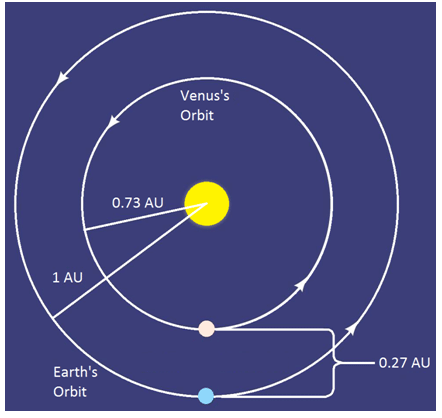However, we could not wait until Venus was at closest approach to Earth to image it. Besides, it is very challenging to image Venus at closest approach to Earth because it is very close to the sun in the sky and its phase is very close to new.

Instead, we imaged Venus when its phase was a waxing gibbous.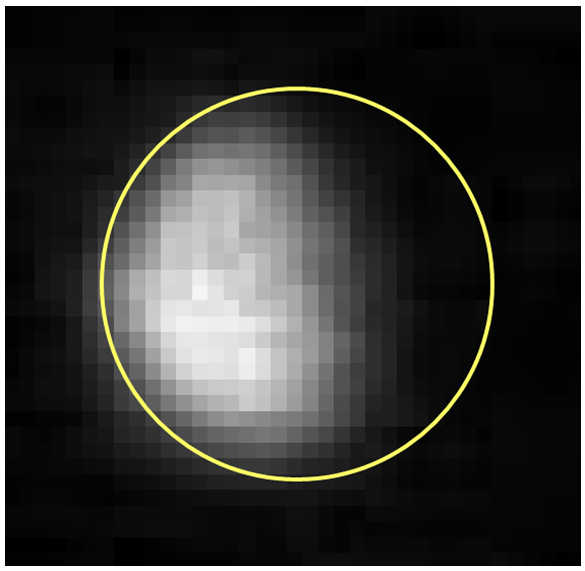Venus can only be a waxing gibbous of this phase when it is in a particular location in its orbit around the sun relative to Earth.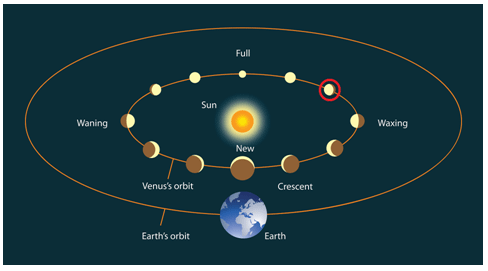This particular location is 1.34 AU from Earth.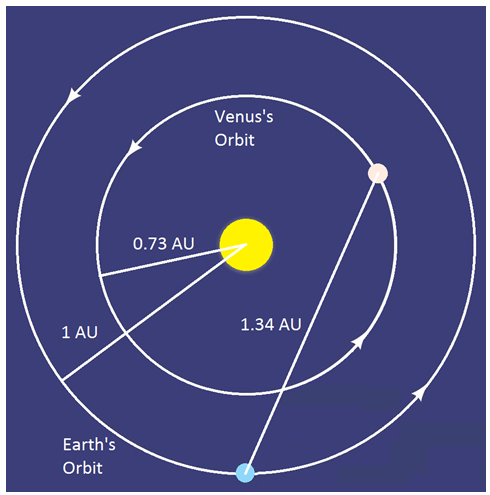We took simultaneous images of Venus from CTIO in Chile and from Morehead Observatory in North Carolina. Since Venus is so bright, we had to use a special filter that blocks most of the light in the center of the image but very little light elsewhere in the image. This way, we could image for long enough to see stars in the images, which are needed to overlay the two images, without saturating Venus, which is needed to measure its angular shift due to parallax.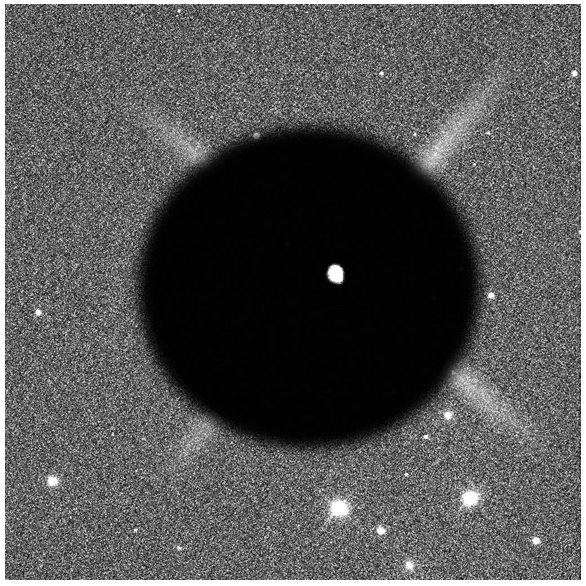These filters are no longer on the telescopes, so we will use the images that we took previously. You can access them under "Sample Images", "Astro 101 lab", "Lab 4 - Parallax", "Venus".

Question: Use Afterglow to align and overlay these images and measure the angular shift of Venus between the images due to parallax, to the nearest 0.1 arcseconds. (1 point)
arcsec

Question: Calculate the baseline between the two observing positions using Earth's true diameter. (1 point each answer box)
baseline = fraction of diameter x true diameter
 fraction of diameter baseline km

Question: Calculate the distance to Venus using parallax. (2 points)
distancebaseline 2πx360 deg θNote: Remember to convert θ to degrees before using this equation!
km

Now, since:
1.34 AU=distance
1 AU =  distance 1.34

Question: Calculate how many kilometers are in one AU using your distance to Venus. (1 point)
km

Question: The true value is 1.5 x 108 km. Calculate your percent error. (1 point)
%

Question: Discuss significant sources of error. (1 point)
• ## PROCEDURE: D. DISTANCE TO ALPHA CENTAURI

Finally, we are going to measure the distance to Alpha Centauri using stellar parallax. Actually, Alpha Centauri consists of two stars - A and B - in close proximity to each other.

Like Venus, Alpha Centauri is sufficiently bright that we had to use one of our special filters to detect other stars without saturating Alpha Centauri.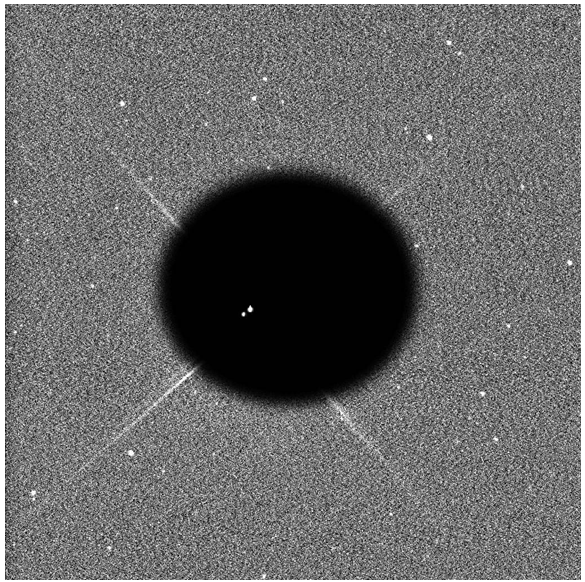Again, since these filters are no longer on the telescopes we will use images that we took previously. You can access them under "Sample Images", "Astro 101 lab", "Lab 4 - Parallax", "Alpha Centauri".

Question: Use Afterglow to align and overlay these images and measure the angular shift of Alpha Centauri between the images due to parallax, to the nearest 0.1 arcseconds. (1 point)
arcsec

Question: These images were taken six months apart, first when Earth was as far to one side of Alpha Centauri as it can get and again when Earth was as far to the other side of Alpha Centauri as it can get. Consequently, the baseline between the two observing positions is how many AU across? (1 point)
AU

Question: First, convert this to kilometers using your measurement of how many kilometers are in an AU. (1 point)
km

Question: Now convert the baseline to kilometers using the true value. (1 point)
km

Question: Calculate the distance to Alpha Centauri using parallax and the true baseline in kilometers. (2 points)
distancebaseline 2πx360 deg θNote: Remember to convert θ to degrees before using this equation!
km

Question: Google and record the true value. (1 point)
km

Question: Calculate your percent error. (1 point)
%

Question: Discuss significant sources of error. (1 point)